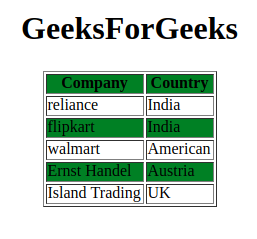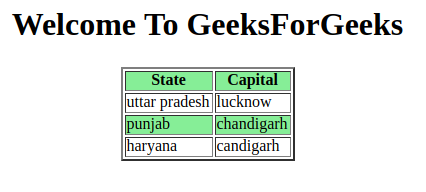# jQuery :even Selector

jQuery is an open-source JavaScript library that simplifies the interactions between an HTML/CSS document, or more precisely the Document Object Model (DOM), and JavaScript. Elaborating on the terms, it simplifies HTML document traversing and manipulation, browser event handling, DOM animations, Ajax interactions, and cross-browser JavaScript development.

The jQuery:even selector used to select an even number index from the elements. The number of the index starts from 0. The working is the same as odd selectors but for even numbers.

Syntax

`\$(":even")`

Example 1:  In this example we are using the above-explained method.

## HTML

 `` `<``html``>`   `<``head``>` `    ``<``script` `src``=` `"https://ajax.googleapis.com/ajax/libs/jquery/3.3.1/jquery.min.js"``>` `    ``` `    ``<``script``>` `        ``\$(document).ready(function () {` `            ``\$("tr:even").css("background-color",` `                ``"green");` `        ``});` `    ``` ``   `<``body``>` `    ``<``center``>` `        ``<``h1``>GeeksForGeeks` `        ``<``table` `border``=``"1"``>` `            ``<``tr``>` `                ``<``th``>Company` `                ``<``th``>Country` `            ``` `            ``<``tr``>` `                ``<``td``>reliance` `                ``<``td``>India` `            ``` `            ``<``tr``>` `                ``<``td``>flipkart` `                ``<``td``>India` `            ``` `            ``<``tr``>` `                ``<``td``>walmart` `                ``<``td``>American` `            ``` `            ``<``tr``>` `                ``<``td``>Ernst Handle` `                ``<``td``>Austria` `            ``` `            ``<``tr``>` `                ``<``td``>Island Trading` `                ``<``td``>UK` `            ``` `        ``` `    ``` ``   ``

Output:Example 2:

## HTML

 `` `<``html``>`   `<``head``>` `    ``<``script` `src``=` `"https://ajax.googleapis.com/ajax/libs/jquery/3.3.1/jquery.min.js"``>` `    ``` `    ``<``script``>` `        ``\$(document).ready(function () {` `            ``\$("tr:even").css("background-color",` `                ``"lightgreen");` `        ``});` `    ``` ``   `<``body``>` `    ``<``center``>` `        ``<``h1``>Welcome To GeeksForGeeks` `        ``` `        ``<``table` `border``=``"2"``>` `            ``<``tr``>` `                ``<``th``>State` `                ``<``th``>Capital` `            ``` `            ``<``tr``>` `                ``<``td``>uttar pradesh` `                ``<``td``>lucknow` `            ``` `            ``<``tr``>` `                ``<``td``>punjab` `                ``<``td``>chandigarh` `            ``` `            ``<``tr``>` `                ``<``td``>haryana` `                ``<``td``>chandigarh` `            ``` `        ``` `    ``` ``   ``

Output:Supported Browsers: